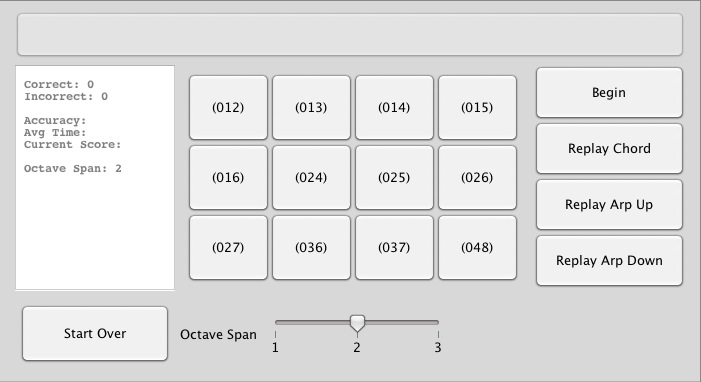Top

Archive of articles classified as "Music Theory"

Back home

## Post-Tonal Ear Training Suite

Click on image to run PTETSuite.## SetCalc

SetCalc is a simple set of tools for pitch-class set analysis, designed to be used on some sort of touch screen device, such as the iPhone, iPad, etc.

Click on image to open SetCalc.## Music Calculators

### SetCalc

SetCalc for mobile devices
Simple set of tools for pitch-class set analysis, designed specifically for iOS.

### plot-pc-set.js

plot-pc-set.js is a script written in javascript that graphs pitch-class sets on a graph, and that animates transpositions and inversions. It is also located as a repository on github.com.

### Other Set Calculators

Set class calculator
Given certain pitch classes in a given modulus, this program calculates the set class, the interval and TICS vectors, the complement, the Fourier partition, and finds z-related sets if any.

Draggable set classes
This page is a sandbox for playing around with set-class graphs, like the graphs made by the set-class calculator. On this page, you can create multiple graphs and move them around for comparison, being useful perhaps for demonstrations and for teaching.

Subset calculator
List all the subsets of a given set in a given modulus.

## Perl Setclass Calculator Script

The following script is entirely my own, for better or for worse. It is a self-contained script that calculates the set class of a given set. Given a particular set of pcs (numbers) in a given mod, the program returns the Tn and TnI classes, and the interval vector. The program is quite powerful, in that it handles decimals, negative numbers and multisets.

To install the script, go to Terminal (in mac), and find the directory where the script is located (using cd). If you need help with that, here is a page outlining some of the basic unix commands.

## bin2pcs Script

This perl script takes a decimal number and converts it to a list of pitch classes, and prints the list to the screen. For example, given the decimal number 145, the corresponding set would be {0, 4, 7}, since 145 = 20 + 24 + 27.

To install, use the same method as given here. Once installed, use the script by doing:

```bin2pcs 145
```